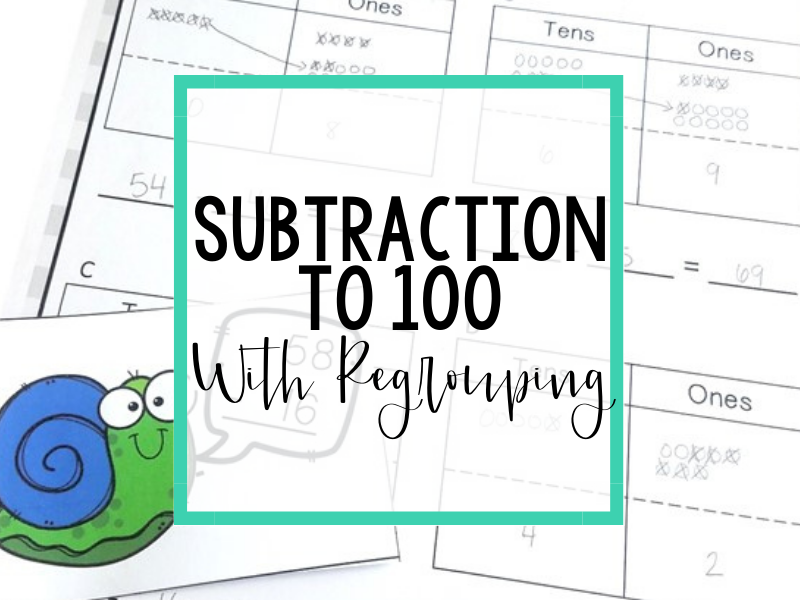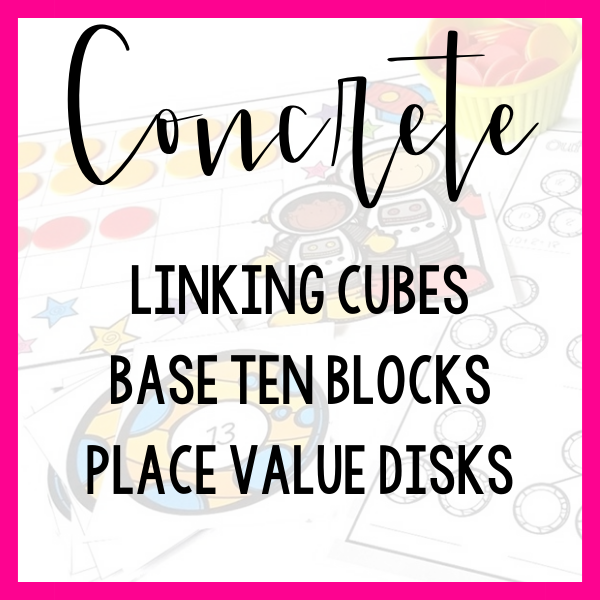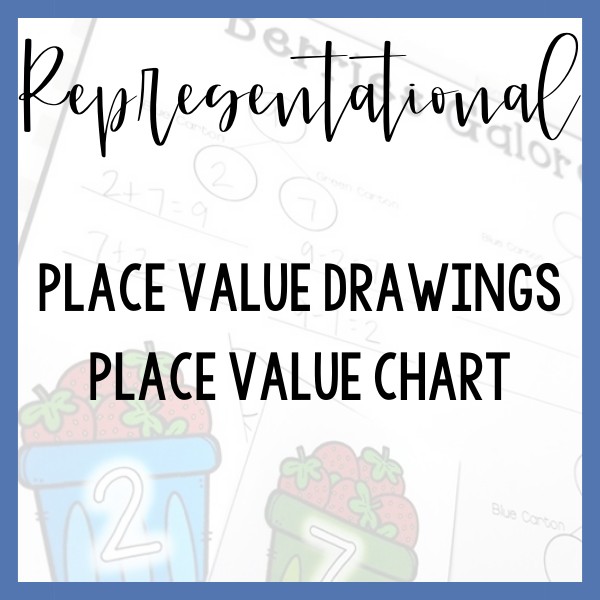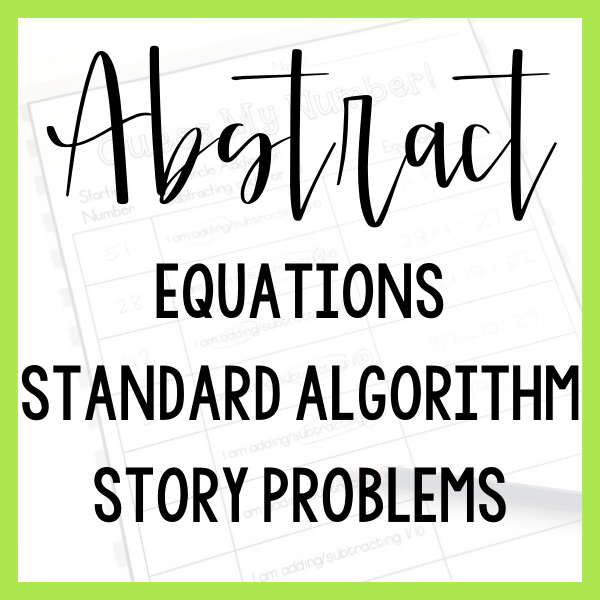# subtraction to 100 with regrouping

It is important to note that 2nd-grade students do not need to master the written standard algorithm for subtracting numbers to 100 let alone 1,000! In fact, students only need to relate a place value strategy to a written method. Start with a focus on supporting your students in subtracting 2-digit numbers using hands-on materials and place value drawings and then relate these representations to a written method.The 5 examples listed are certainly not an exhaustive list of tools and lessons that could be used to teach this skill.

They are rather a sample progression from hands-on to abstract thinking!

Which step represents your students’ current level of understanding?

Linking cubes are a groupable model. This means that as your students decompose a ten, they can physically take their cubes apart creating ten ones.

Support your students futher by asking them to model subtraction with linking cubes on a place value chart.

Because you have the goal of ultimately relating this place value strategy to the written algorithm, you will want your students to organize their linking cubes on a place value chart in the same vertical configuration that they will ultimately use when following the standard algorithm for subtraction.

Frequently ask your students to relate their model to the meaning of subtraction- How is this model helping us to start with a whole and take it away, apart or find the difference?

You will want to support your students in moving from a groupable model (linking cubes) to a pre-grouped model such as base ten blocks (proportional) or place value disks (non-proportional).

In moving toward a pre-grouped model, you are still supporting your students with a concrete, hands-on experience but you are lessening the support provided by the manipulative in order to move your students towards abstract thinking.

Think of this as taking baby steps away from hands-on materials.

When using a pre-grouped model, continue to arrange your materials on a place value chart to mimick the ultimate organization of the standard algorithm.

Introduce the standard algorithm directly alongside hands-on materials that your students have been successful in using.

As your students model with hands-on materials, think aloud and reflect back the actions they have taken, one step at a time, and record their thinking using the standard algorithm.

For example: You wanted to take away the 3 ones in 23 from the 1 one in 41 but there weren’t enough ones to take 3 away. You decomposed a ten into ten ones. Let’s show that in our vertical equation by crossing off a ten and adding ten more ones to the ones column.

As you complete problems, ask questions of your students that require them to link their understanding of hands-on materials with the written method.

• I see you built 41 using place value disks. Where do we see the 41 in our equation?
• I see that you decomposed a ten and put the ten ones into the ones column. Where did we show that in our equation?

While your students are now able to relate a hands-on method to a written method, you want to take a step back and move your students from hands-on supports to representative models.

In this case, a place value drawing on a place value chart can be a supportive model for your students.

Begin by asking your students to model a subtraction equation using place value disks. As they are working, record their actions, one step at a time, as a place value drawing on a place value chart.

The process and questioning is very similar to the process for linking hands-on and written methods.

Although your students have now had exposure to subtraction with regrouping using hands-on materials, place value drawings and the written algorithm, you have not explicitly linked the representative drawing with the written algorithm- don’t skip this step!

Think of your students understanding of math concepts as a large web. Each strategy, method and skill is it’s own point on the web. The web is strengthened when these points are linked together.

Just as you used hands-on materials alongside the written method, repeat this process by asking your students to solve using a place value drawing alongside the written method.

Again, focus on asking linking questions that require students to articulate how they subtract using each of these methods.

# Tools To USe